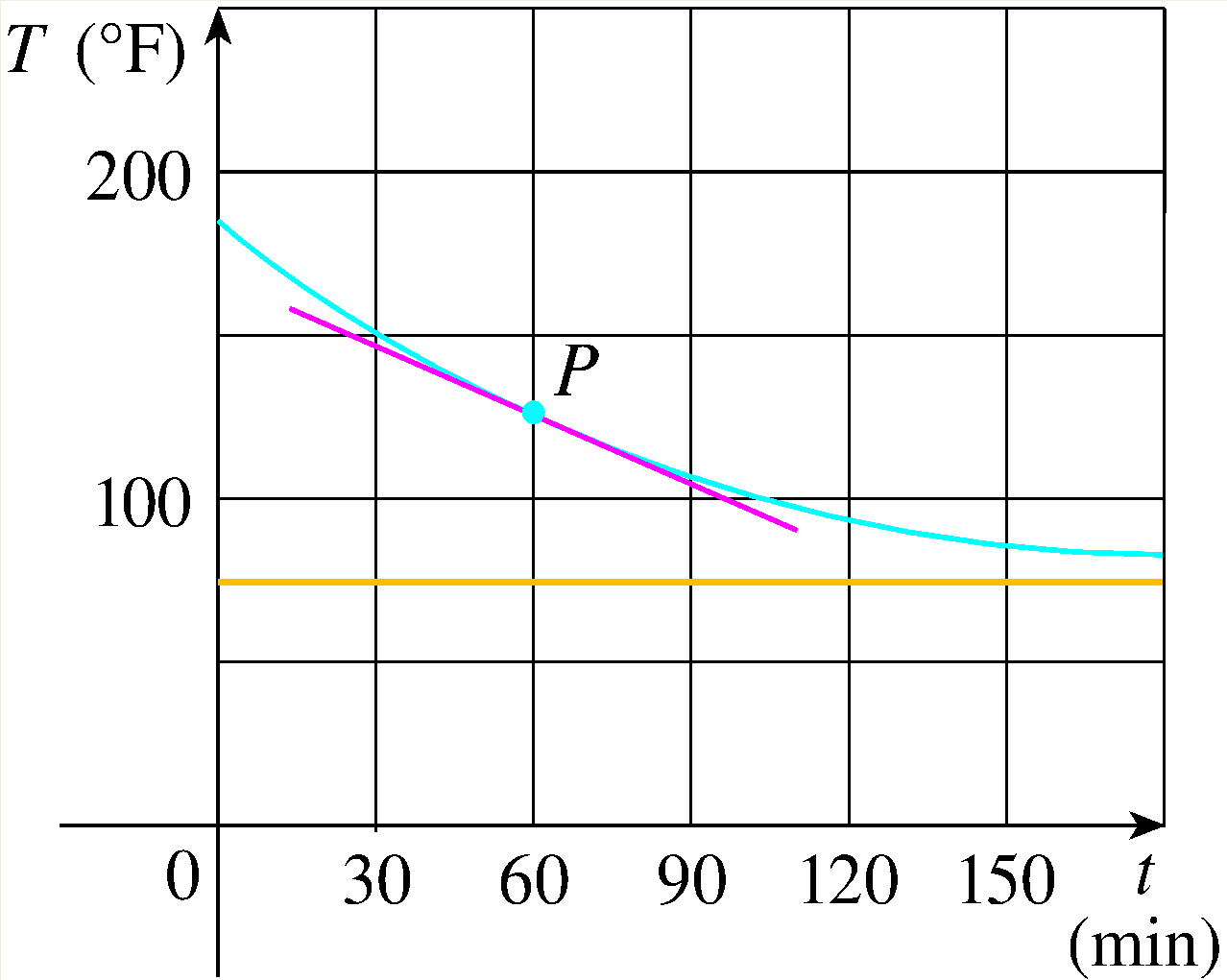A roast turkey is taken from an oven when its temperature has reached $185^{\circ }$F and is placed on a table in a room where the temperature is $75^{\circ }$F.
By measuring the slope of the tangent, estimate the rate of change of the temperature after an hour. Your answer must be within 20% of the correct value.

slope at $P =$